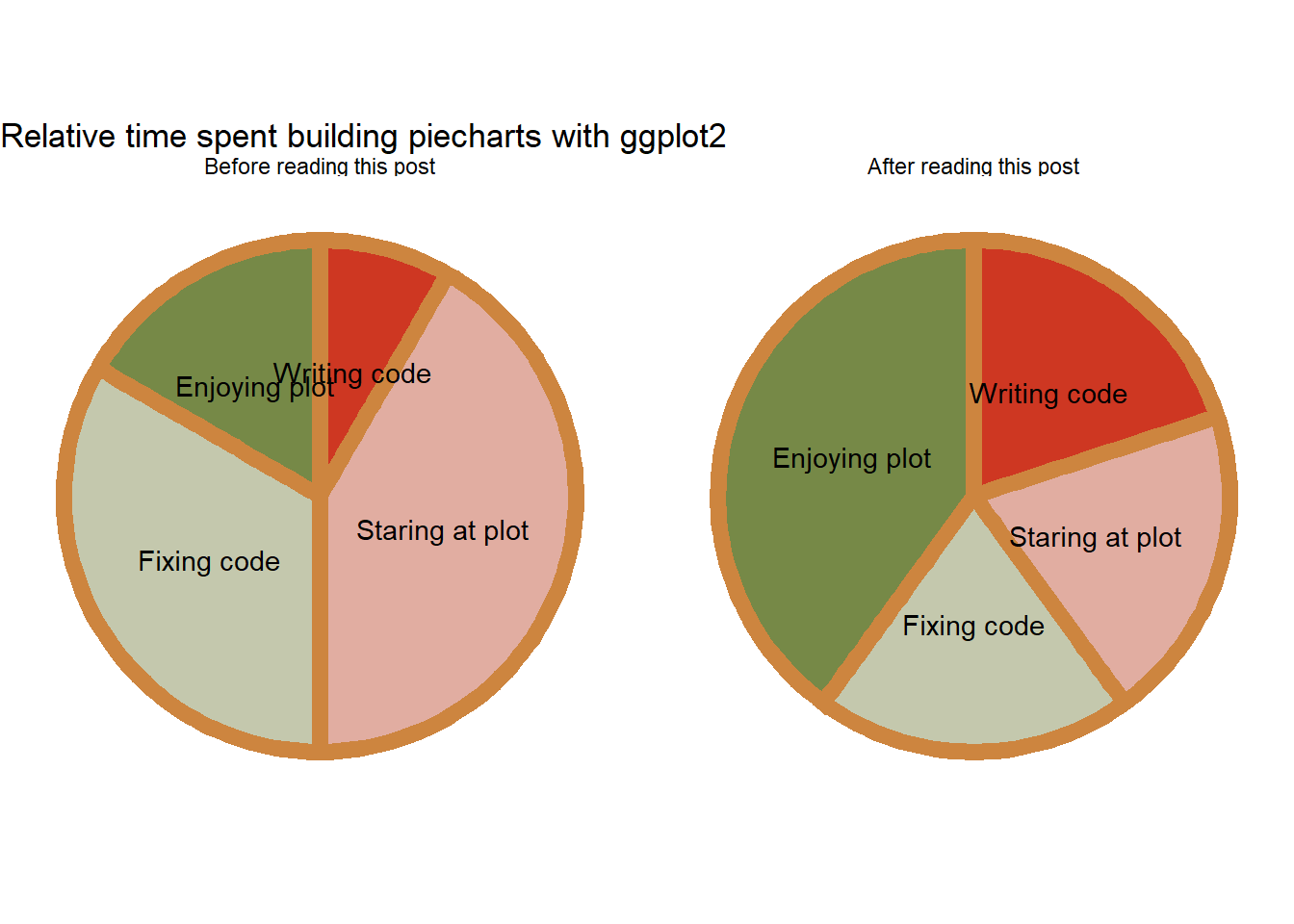# ggplot: Easy as pie (charts)

R
ggplot2
Author

Mattan S. Ben-Shachar

Published

August 12, 2021

This post by no means endorses the use of pie charts. But, if you must, here’s how…

For some reason, the top Google results for “ggplot2 pie chart” show some very convoluted code to accomplish what should be easy:

1. Make slices
2. Add labels to the middle of those slices

Instead, let’s look at the easy way - with `position_stack()`!

### Make some data

We first need some data fit for a pie chart - a column for slice label, and a column for their (preferably relative) size.

``````d <- data.frame(
Slices = c("Writing code", "Staring at plot", "Fixing code", "Enjoying plot") |> rep(2),
Time = c(1, 5, 4, 2, 1, 1, 1, 2),
When = c("Before reading this post", "After reading this post") |> rep(each = 4)
) |>
transform(
# Make time relative
Time_relative = Time / ave(Time, When, FUN = sum),
Slices = factor(Slices, levels = unique(Slices)),
When = factor(When, levels = unique(When))
)

d``````
``````#>            Slices Time                     When Time_relative
#> 1    Writing code    1 Before reading this post    0.08333333
#> 2 Staring at plot    5 Before reading this post    0.41666667
#> 3     Fixing code    4 Before reading this post    0.33333333
#> 4   Enjoying plot    2 Before reading this post    0.16666667
#> 5    Writing code    1  After reading this post    0.20000000
#> 6 Staring at plot    1  After reading this post    0.20000000
#> 7     Fixing code    1  After reading this post    0.20000000
#> 8   Enjoying plot    2  After reading this post    0.40000000``````

### Now let’s build the pie-chart!

``````library(ggplot2)

ggplot(d, aes(x = 1, y = Time_relative, fill = Slices)) +
facet_grid(cols = vars(When)) +
# Make pie
coord_polar(theta = "y") +
geom_col(position = position_stack(reverse = TRUE),
color = "tan3", linewidth = 3, show.legend = FALSE) +
# Add labels to the *stacked* position,
# in the middle of the column (vjust = 0.5)
geom_text(aes(label = Slices),
position = position_stack(vjust = 0.5, reverse = TRUE)) +
# Make it a pizza pie!
see::scale_fill_pizza_d() +
theme_void() +
labs(title = "Relative time spent building piecharts with ggplot2")``````Best served HOT!